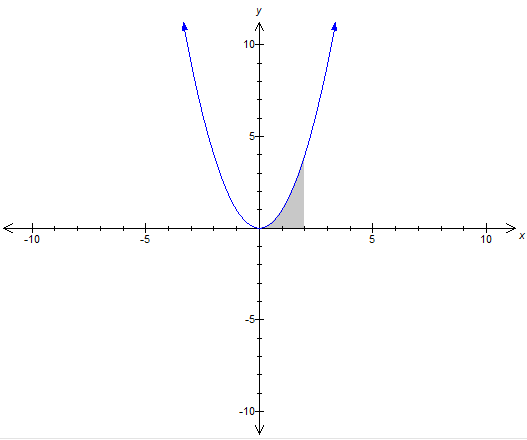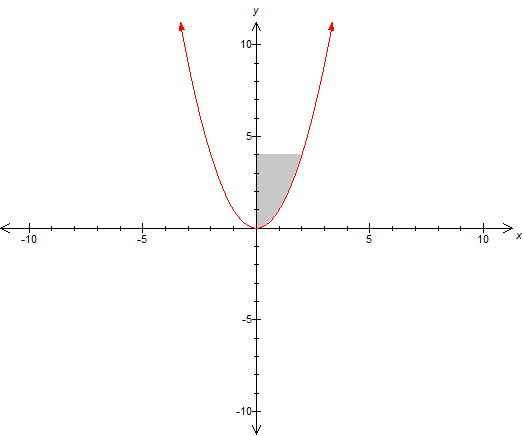# 2 Questions Regarding Integrals

13 replies to this topic

### #1toprun91

toprun91

Newbie

•• Members
•• 4 posts

Posted 24 February 2010 - 02:19 PM

Hi is it possible to find integrals enclosed along the y axis?, and can you find indefinite integrals in the graph mode. Thx

### #2ASTRO491K

ASTRO491K

•• Members
•• 94 posts

Posted 24 February 2010 - 05:25 PM

Integrals enclosed along the y axis? Please explain what you mean by that.

Hi is it possible to find integrals enclosed along the y axis?, and can you find indefinite integrals in the graph mode. Thx

### #3toprun91

toprun91

Newbie

•• Members
•• 4 posts

Posted 25 February 2010 - 12:58 AM

Integrals enclosed along the y axis? Please explain what you mean by that.

What i mean is finding the area enclosed by the function and the y axis

For example for the function y=x^2

If the area is enclosed by the x axis it would be like thisand if the area is enclosed by the y axis it would be like this### #4pan.gejt

pan.gejt

Casio Freak

•• Members
•• 232 posts
• Gender:Male
• Location:CZ

• Calculators:
.

Posted 25 February 2010 - 05:58 AM

1. calculate the rectangular area S = (x2-x1)*(y2-y1)
2. calculate the area under the curve according to Newton' formula- see your first picture
3. subtract these 2 areas for getting the result as seen on your second picture

### #5toprun91

toprun91

Newbie

•• Members
•• 4 posts

Posted 25 February 2010 - 11:05 AM

1. calculate the rectangular area S = (x2-x1)*(y2-y1)
2. calculate the area under the curve according to Newton' formula- see your first picture
3. subtract these 2 areas for getting the result as seen on your second picture

Is there a way to type it into a casio classpad

### #6pan.gejt

pan.gejt

Casio Freak

•• Members
•• 232 posts
• Gender:Male
• Location:CZ

• Calculators:
.

Posted 25 February 2010 - 03:38 PM

1. define the user function (menu - main - interactive - define)
2. store the lower and upper limits to variables- e.g. a and b, this step is optional, you can use numeric input instead of using the variables
3. type [(b-a)*(f(-f(a)] - INT(f(x), a, b, x), where INT is template of definite integral (or use the numeric integration) , f(x) is user-defined function a, b are lower and upper limits
or type directly [(5-1)*(f(5)-f(1)] - INT(f(x), 1, 5, x)
4. press "exe" button
If the result is negative (S<0), use "abs" function to correct this.

edited: why there is a smile instead of function value in point b? test: f(a), f(, f©

Edited by pan.gejt, 25 February 2010 - 03:44 PM.

### #7DanielT

DanielT

Newbie

•• Members
•• 13 posts

• Calculators:
Ti-89 Titanium
Casio fx-9750GII

Posted 25 February 2010 - 05:01 PM

Is there a way to type it into a casio classpad

you can find an inverse of that function and integrate from x = 0 to 4.
In the classpad, you swap x and y. Then you solve for the new y.
Then plug in the function.

### #8ASTRO491K

ASTRO491K

•• Members
•• 94 posts

Posted 26 February 2010 - 02:21 AM

Perhaps what he is after is simply INT(y^2, 1, 5, y)

edited: why there is a smile instead of function value in point b? test: f(a), f(, fÂ©
[/quote]

### #9pan.gejt

pan.gejt

Casio Freak

•• Members
•• 232 posts
• Gender:Male
• Location:CZ

• Calculators:
.

Posted 26 February 2010 - 04:46 AM

Finding the inversion function is not simple and sometimes the inversion function does not exist (in most cases).
Using INT(y^2, 1, 5, y) does not solve the situation, because the integration is still in terms of "x" and not "y"
if the inversion function exists you should recalculate the lower and upper limits and integrate the inversion function
e.g. for function f(x)=x^2 :
lower limit a=1 therefore y(a)=1
upper limit b=5 therefore y(=25
so integration in case of function shown above should be between 1 and 25.

### #10ASTRO491K

ASTRO491K

•• Members
•• 94 posts

Posted 26 February 2010 - 04:25 PM

Perhaps you are right, but it occurred to me that perhaps we are making to much of this thread and all the guy wants to know is, can I find the area between the y-axis and a function x=f(y). I think we need a clarification from the original poster as to what he wants.

Finding the inversion function is not simple and sometimes the inversion function does not exist (in most cases).
Using INT(y^2, 1, 5, y) does not solve the situation, because the integration is still in terms of "x" and not "y"
if the inversion function exists you should recalculate the lower and upper limits and integrate the inversion function
e.g. for function f(x)=x^2 :
lower limit a=1 therefore y(a)=1
upper limit b=5 therefore y(=25
so integration in case of function shown above should be between 1 and 25.

### #11toprun91

toprun91

Newbie

•• Members
•• 4 posts

Posted 26 February 2010 - 11:21 PM

Thx guys, but a little confused, i am not that pro at maths

### #12Guest_VasiLisa_*

Guest_VasiLisa_*
•• Guests

Posted 02 January 2011 - 12:23 PM

Всех с Новым годом!
Я новичок. Помогите кто знает, как загрузить картинку (UserBar) в подпись?
Никак не пойму.
Как я поняла, здесь можно загрузить картинку только по ссылке на другом сайте, а как загрузить ее со своего компьютера?
У меня есть красивая картинка-UserBar на компьютере, хочу его закачать, но не понимаю как...

### #13JosJuice

JosJuice

•• Members
•• 75 posts
• Gender:Not Telling
• Location:Sweden

• Calculators:
Casio Prizm fx-CG10
TI-84 Plus Silver Edition

Posted 02 January 2011 - 03:17 PM

Всех с Новым годом!
Я новичок. Помогите кто знает, как загрузить картинку (UserBar) в подпись?
Никак не пойму.
Как я поняла, здесь можно загрузить картинку только по ссылке на другом сайте, а как загрузить ее со своего компьютера?
У меня есть красивая картинка-UserBar на компьютере, хочу его закачать, но не понимаю как...

You need to upload it to some kind of website. You can't upload it directly from your computer to the forum - that can only be done with avatars.
Also, you're in the wrong topic (and in the wrong language...) I had to use an automatic translator to read, so my answer may not be completely relevant to your question.

### #14Guest_VasiLisa_*

Guest_VasiLisa_*
•• Guests

Posted 02 January 2011 - 08:16 PM

Ок, похоже поняла.
Всем спасибо за помощь#### 0 user(s) are reading this topic

0 members, 0 guests, 0 anonymous users